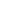# Free Unit 3-Coordinate Geometry Worksheets

Download free printable Unit 3-Coordinate Geometry Worksheets to practice. With thousands of questions available, you can generate as many Unit 3-Coordinate Geometry Worksheets as you want.

## Sample Unit 3-Coordinate Geometry Worksheet Questions

1.

What is the length of the line segment whose end points are A (1,-4) and B (5, -7).

1.

√157 units

2.

5 units

3.

√45 units

4.

√137 units

2.

If C(-5,0) is the midpoint of the line segment AB with A at (3, -1), what are the coordinates of point B?

1.

(-1, -0.5)

2.

(-7, 1)

3.

(-7, -1)

4.

(-13, 1)

3.

We can find whether any 3 given points are collinear using the distance formula.

1. True 2. False
4.

We can find whether any given points are collinear or not using the distance formula.

1. True 2. False
5.

If the value of abscissa of a point A is 0, where does the A lie on?

1.

Origin

2.

X-axis

3.

Y-axis

4.

None of the above

6.
Choose the correct option: Point (-9, 0) lies on _______.
1. on the negative direction of the x-axis 2. on the negative direction of the y-axis 3. I quadrant 4. II quadrant
7.
Choose the correct option: If y coordinate of a point is zero, then this point always lies on _______.
8.
Choose the correct option: Signs of the abscissa and ordinate of a point in the second quadrant are respectively ________.
1. (+, +) 2. (–, –) 3. (+, –) 4. (–, +)
9.
Choose the correct option: The points whose abscissa and ordinate have different signs will lie in _________.
10.
Choose the correct option: Abscissa of a point is negative in ________.

Worksheets by UrbanPro

Our worksheets are designed to help students explore various topics, practice skills and enrich their subject knowledge, to improve their academic performance. Designed by Experts who have extensive experience and expertise in teaching a subject, these worksheets will improve your child's problem-solving skills and subject knowledge in a fun and interactive manner.
Check out our free customized worksheets across school boards, grades, subjects and levels of subject knowledge. You can download, print and share these worksheets with anyone, anywhere, anytime!

Get a custom worksheet to practice!

Select your topic & see the magic.

subjectSelect Chapter(s)

Chapters & Subtopics